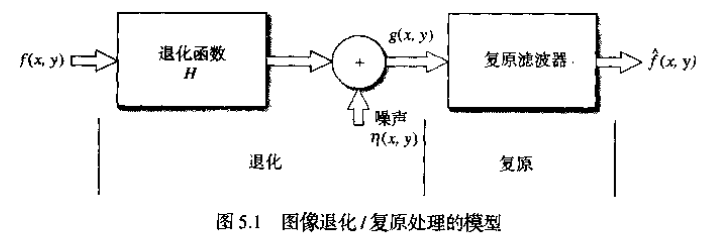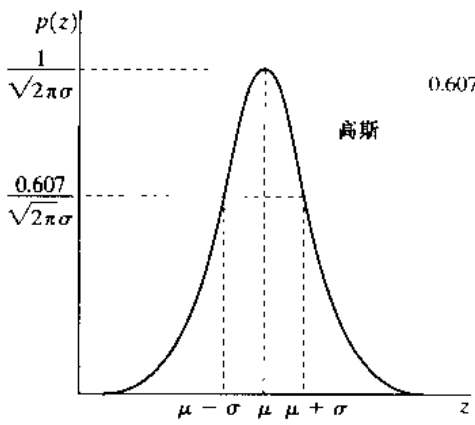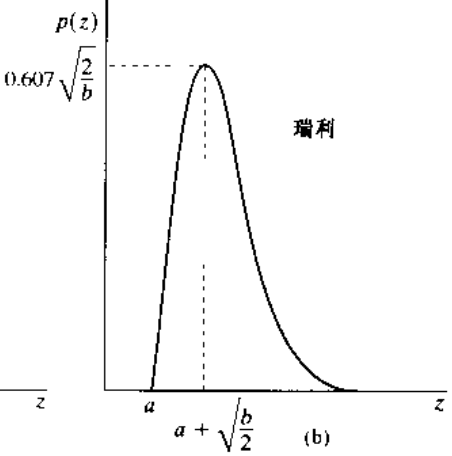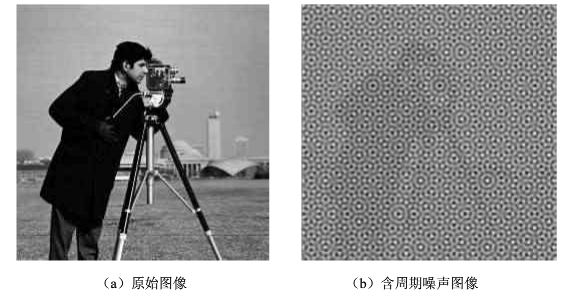# 数字图像处理笔记—图像复原（一）

## 1 图像退化/复原过程模型g(x,y)=h(x,y)*f(x,y)+η(x,y)

G(u,v)=H(u,v)F(u,v)+N(u,v)

## 2 噪声模型

### 2.2 一些重要的噪声概率密度函数（PDF）

1. 高斯噪声
概率密度函数： p(z)=12πσezμ)2/2σ2 $p(z)=\frac{1}{\sqrt{2\pi}\sigma}e^{-（z-\mu)^2/2\sigma^2}$
均值： μ $\mu$
方差： σ2 $\sigma^2$
曲线：2. 瑞利噪声
概率密度函数：
p(z)={2b(za)e(za)2/b,0,zaz<a $p(z)=\begin{cases}\frac{2}{b}(z-a)e^{-(z-a)^2/b},&z\ge a\\0,&z
均值： μ=a+πb/4 $\mu=a+\sqrt{\pi b}/4$
方差： σ2=b(4π)4 $\sigma^2=\frac{b(4-\pi)}{4}$
曲线：3.伽马（爱尔兰）噪声
p(z)=abz(b1)(b1)!eaz,0,z0z<0 $p(z)=\begin{cases}\frac{a^bz^(b-1)}{(b-1)!}e^{-az},&z\ge 0\\0,&z<0\end{cases}$

4. 指数分布噪声

p(z)={aeaz,0,z0z<0 $p(z)=\begin{cases}ae^{-az},&z\ge 0\\0,&z<0\end{cases}$

5. 均匀噪声

6. 脉冲（椒盐）噪声

### 2.3 周期噪声

1.来源：主要是在图像获取过程中从电力或机电干扰中产生的。
2.是唯一的一种空间依赖性噪声（即该噪声不是对原图像某一区域的影响，而是对图像的整个空域都有影响）。空间上表现为周期性，在频域中是孤立的点，因此常用频域的办法来滤除周期噪声3.噪声参数估计

## 3 噪声存在下的唯一空间滤波

当图像中的退化仅有加性噪声时，即退化模型变为下式这样


### 3.1 均值滤波器

• 算术均值滤波器
描述： f^(x,y)=(s,t)Sxyg(s,t) $\hat{f}(x,y)=\sum_{(s,t)\in S_{xy}}g(s,t)$
结果：模糊了结果，降低了噪声
适用：适用于高斯噪声或均匀随机噪声

• 几何均值滤波器
描述： f^(x,y)=[(s,t)Sxyg(s,t)] $\hat{f}(x,y)=\left [ \prod_{(s,t)\in S_{xy}}g(s,t) \right ]$
结果：和算术均值滤波器相比，丢失更少的细节
适用：同算术均值滤波器

• 谐波均值滤波器
描述： f^(x,y)=mn(x,y)Sxy1g(s,t) $\hat{f}(x,y)=\frac{mn}{\sum_{(x,y)\in S_{xy}}\frac{1}{g(s,t)}}$
结果：谐波均值滤波器对于“盐”噪声效果更好，但是不适用于“胡椒”噪声。
适用：像高斯那样的噪声

• 逆谐波均值滤波器
描述： f^(x,y)=(s,t)Sxyg(s,t)Q+1(s,t)Sxyg(s,t)Q $\hat{f}(x,y)=\frac{\sum_{(s,t)\in S_{xy}}g(s,t)^{Q+1}}{\sum_{(s,t)\in S_{xy}}g(s,t)^Q}$
其实Q称为滤波器的阶数，当Q值为正时，滤波器用于消除“胡椒”噪声；当Q值为负时，滤波器用于消除“盐”噪声。但它不能同时消除两种噪声，当Q值为0时，逆谐波滤波器变为算术均值滤波器；当Q为-1时，逆谐波均值滤波器退变为谐波均值滤波器。
适用：脉冲噪声
缺点：必须知道是明噪声还是暗噪声

### 3.2 顺序统计滤波器

- 中值滤波器

• 最大值滤波器
描述： f^(x,y)=max(s,t)Sxy{g(s,t)} $\hat{f}(x,y)=\max_{(s,t)\in S_{xy}}\{g(s,t)\}$
结果：
适用：发现图像中的最亮点，故适用于去除“胡椒”噪声

• 最小值滤波器
描述： f^(x,y)=min(s,t)Sxy{g(s,t)} $\hat{f}(x,y)=\min_{(s,t)\in S_{xy}}\{g(s,t)\}$
适用：发现图像中的最暗点，适用于去除“盐”噪声

• 中点滤波器
描述： f^(x,y)=[max(s,t)Sxy{g(s,t)}+min(s,t)Sxy{g(s,t)}] $\hat{f}(x,y)=\left [ \max_{(s,t)\in S_{xy}}\{g(s,t)\}+ \min_{(s,t)\in S_{xy}}\{g(s,t)\}\right ]$
适用：结合了顺序统计和求平均，对高斯和均匀随机分布的噪声有很好的效果

• 修正后的阿尔法均值滤波器
描述： f^(x,y)=1mn(s,t)Sxy{gr(s,t)} $\hat{f}(x,y)=\frac{1}{mn}\sum_{(s,t)\in S_{xy}}\{ g_r (s,t)\}$
Sxy $S_{xy}$邻域内取点g（s，t）最高灰度值的d/2和最低灰度值的d/2，用 gr(s,t) $g_r(s,t)$来代表剩余的mn-d个像素，由这些剩余像素点的平均值形成的滤波器称为修正后的阿尔法均值滤波器
当d=0时，退变为算术均值滤波器；当d=（mn-d）/2时，退变为中值滤波器。
适用：对多重混合的噪声有很好的效果

### 3.3 自适应滤波器

• 自适应、局部噪声消除滤波器
描述： f^(x,y)=g(x,y)σ2ησ2L[g(x,y)mL] $\hat{f}(x,y)=g(x,y)-\frac{\sigma^2_\eta}{\sigma^2_L}[g(x,y)-m_L]$
其中
σ2η $\sigma^2_\eta$ g(x,y) $g(x,y)$形成的噪声方差
mL $m_L$，局部像素点的均值
σ2L $\sigma^2_L$，局部方差
滤波器性能预期：
（1）如果 σ2η $\sigma^2_\eta$为零，滤波器应该简单的返回g（x，y）的值。
（2）如果局部方差与 σ2η $\sigma^2_\eta$是高度相关的，那么滤波器要返回一个g（x，y）的近似值。
（3）如果两个方差相等，希望滤波器返回区域Sxy上像素的算术均值。
一个关键的问题是 σ2η $\sigma^2_\eta$未知，需要进行估计，合理的估计会带来较好的滤波效果。
适用：防止由于缺乏图像噪声方差知识而产生的无意义结果，适用均值和方差确定的加性高斯噪声。
• 自适应中值滤波器
描述：自适应中值滤波算法分两层进行
先考虑如下符号：
Zmin=Sxy中灰度值的最小值
Zmax=Sxy中灰度值的最大值
Zmed=Sxy中灰度值的中间值
Zxy=在坐标（x，y）上的灰度值
Smax=Sxy允许的最大尺寸
算法流程：
A层： A1=Zmed-Zmin；
A2=Zmed-Zmax；
如果A1>0且A2<0（即Zmin

04-161530
04-18114209-022万+
06-091142
07-27846
11-103万+
04-181793
04-14
09-295294
05-141256
07-232万+
01-052万+
06-06
06-26297
02-245861
06-28836
12-02992
04-251万+
08-095396